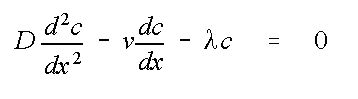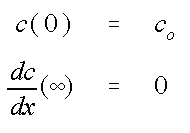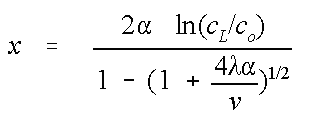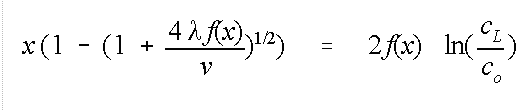# EPA On-line Tools for Site Assessment Calculation

Plume Length Estimate
See bottom of page for equations solved.

Seepage Velocity (qs = v)

mg/L

##### QA check (Direct Solution--Fixed Dispersivity)

The direct solution should match any of the iterative solution results with the same dispersivity. Enter a the fixed dispersivity of interest above to match cases 1, 2 or 3.

Plume Length for Steady One-Dimensional Transportc = concentration [m/L -3 ]
t = time [T]
x = distance [L]
D = dispersion constant [L 2 /T]
v = seepage velocity [L/T]
λ = first order decay constant [1/T]

with the initial and boundary conditionsHas the solution (rearranged for length, x):where the dispersion coeffeicient D, has been separated into a dispersivity, α and the seepage velocity v, and
c L is the concentration that defines the plume length.
i.e., to find the distance (plume length) to the 0.02 mg/L contour, c L is set to 0.02.

Allowing for the dispersivity to be a function of x, f(x):The calculator has three options for f(x):

• Fixed Dispersivity
• One-tenth the plume Length
• Xu and Eckstien (1995) regression relation for plume length (L p = x):